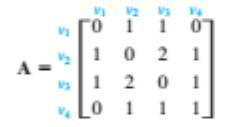Chapter 10.2, Problem 20ES### Discrete Mathematics With Applicat...

5th Edition
EPP + 1 other
ISBN: 9781337694193

#### Solutions

Chapter
Section### Discrete Mathematics With Applicat...

5th Edition
EPP + 1 other
ISBN: 9781337694193
Textbook Problem
1 views

# The following is an adjacency matrix for a graph:Answer the following questions by examining the matrix and its powers only, not by drawing the graph:How many walks of length 2 are there from v2to v3? How many walks of length 3 are there from v1 to v4? How many walks of length 3 are there from v2 to v3?

To determine

(a)

How many walks of length 2 are there from v2 to ν3ν

Explanation

Given information:

A adjacency matrix for a graph:

v 1 v 2 v 3 v 4 v 1 v 2 v 3 v 4 [ 0 1 1 0 1 0 2 1 1 2 0 1 0 1 1 1 ]

Calculation:

Let A=.

The ijth element in A2 represents the number of walks from vi to vj of length 2.The first matrix A has dimensions 2×2 and the second matrix A has dimension 2×2. Since the inner dimensions are equal, we can multiply the matrices. Moreover, the product should be a 2×2-matrix.

Multiply the rows of the first matrix with the columns of the second matrix (adding the products of the corresponding elements):

A 2 =AA=[ 0 1 1 0 1 0 2 1 1 2 0 1 0 1 1 1 ][ 0 1 1 0 1 0 2 1 1 2 0 1 0 1 1 1 ]

=[ 00+11+11+00 01+10+12+01 01+12+10+01 00+11+11+01 10+01+21+10

To determine

(b)

How many walks of length 2 are there from v3 to v4 ?

To determine

(c)

How many walks of length 3 are there from v1 to v4 ?

To determine

(d)

How many walks of length 3 are there from v2 to v3 ?

### Still sussing out bartleby?

Check out a sample textbook solution.

See a sample solution

#### The Solution to Your Study Problems

Bartleby provides explanations to thousands of textbook problems written by our experts, many with advanced degrees!

Get Started